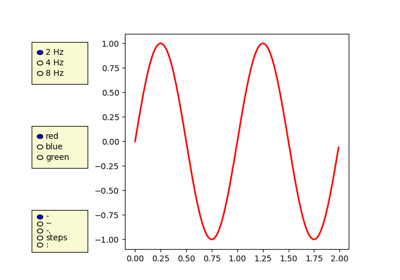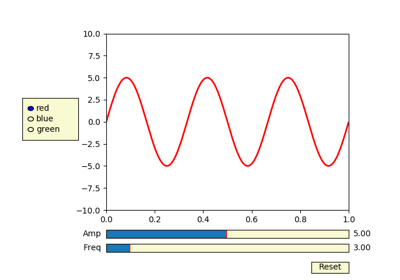Travis-CI:# matplotlib.pyplot.axes¶

`matplotlib.pyplot.``axes`(*args, **kwargs)

Add an axes to the figure.

The axes is added at position rect specified by:

• `axes()` by itself creates a default full `subplot(111)` window axis.
• `axes(rect, facecolor='w')` where rect = [left, bottom, width, height] in normalized (0, 1) units. facecolor is the background color for the axis, default white.
• `axes(h)` where h is an axes instance makes h the current axis and the parent of h the current figure. An `Axes` instance is returned.
kwarg Accepts Description
facecolor color the axes background color
frameon [True|False] display the frame?
sharex otherax current axes shares xaxis attribute with otherax
sharey otherax current axes shares yaxis attribute with otherax
polar [True|False] use a polar axes?
aspect [str | num] [‘equal’, ‘auto’] or a number. If a number the ratio of x-unit/y-unit in screen-space. Also see `set_aspect()`.

Examples:

• `examples/pylab_examples/axes_demo.py` places custom axes.
• `examples/pylab_examples/shared_axis_demo.py` uses sharex and sharey.

## Examples using `matplotlib.pyplot.axes`¶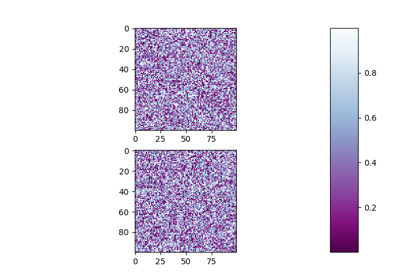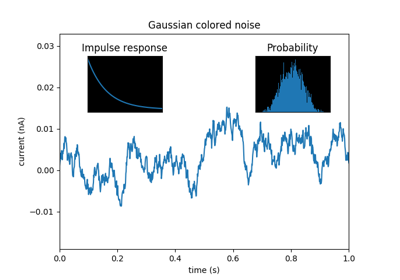Axes Demo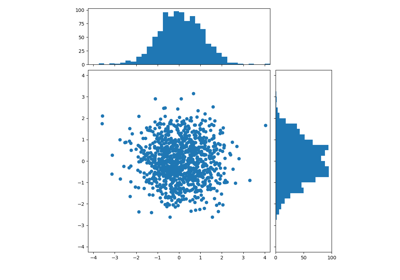Scatter Hist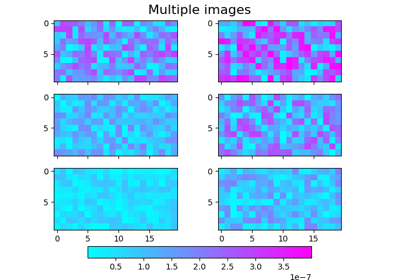Multi Image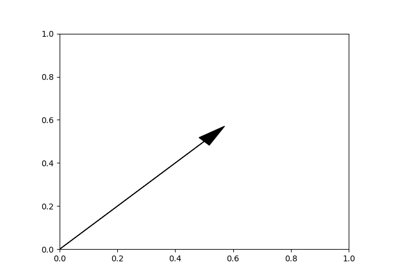Arrow Simple Demo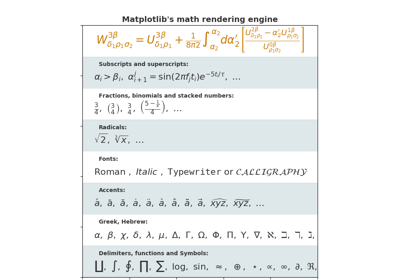Mathtext Examples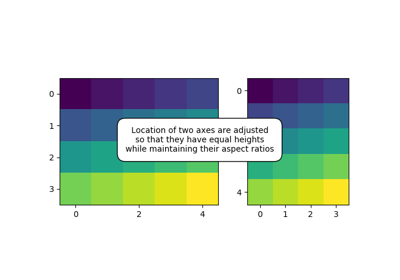Demo Axes Hbox Divider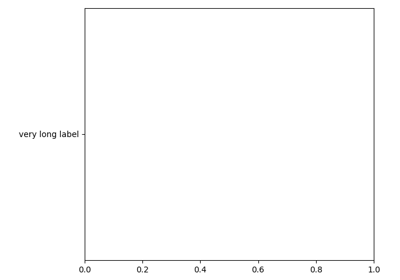Make Room For Ylabel Using Axesgrid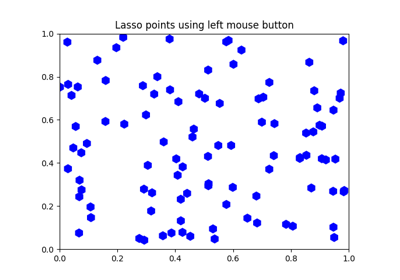Lasso Demo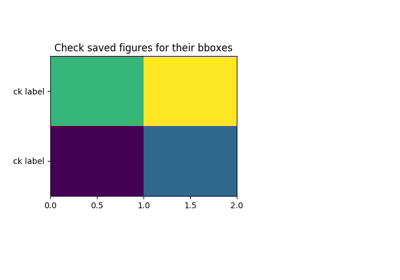Tight Bbox Test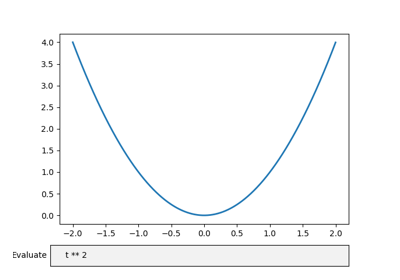Textbox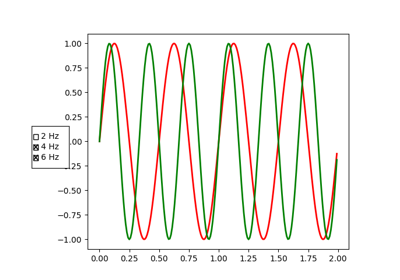Check Buttons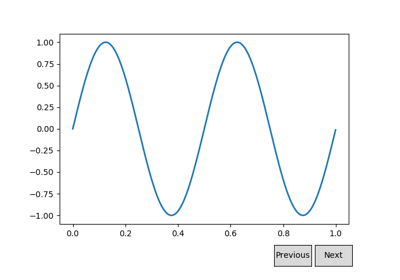Buttons